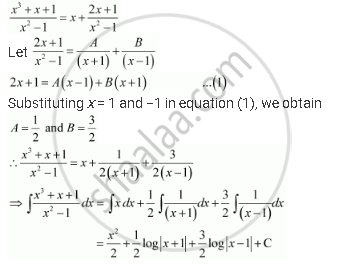Share

# Integrate the Rational Functions (X^2 + X + 1)/(X^2 -1) - CBSE (Science) Class 12 - Mathematics

ConceptMethods of Integration - Integration Using Partial Fractions

#### Question

Integrate the rational functions (x^2 + x + 1)/(x^2 -1)

#### Solution

It can be seen that the given integrand is not a proper fraction.

Therefore, on dividing (x3 + x + 1) by x2 − 1, we obtainIs there an error in this question or solution?

#### Video TutorialsVIEW ALL 

Solution Integrate the Rational Functions (X^2 + X + 1)/(X^2 -1) Concept: Methods of Integration - Integration Using Partial Fractions.
S